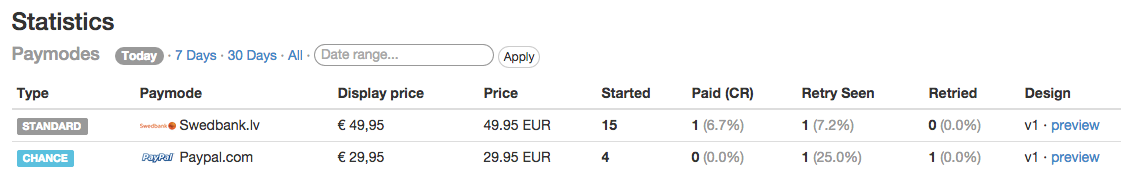# Deep nested queries in ActiveRecord

Contributed the backend of a statistics panel today.Wrote complex, deep-nested queries for associated object data in Activerecord, for example

``````@base_query = Payment.joins(order: {page: :window}).in_daterange(@start_date, @end_date).where("windows.id = ?", window.id).where("provider = ?", @payment_system.provider)
``````As you can see, I use a custom scope `in_daterange(@start_date, @end_date)` that takes two arguments. Here is the definition

``````scope :in_daterange, ->(start_date, end_date) { where(created_at: start_date.to_date.beginning_of_day..end_date.to_date.end_of_day) }
``````

For calculating percentages, handling 0/0 cases is important, here is a helper for that

``````def calculate_percentage divisible, divisor
return (divisible.to_f / (divisor.to_f + Float::EPSILON) * 1000).ceil / 10.0
end
``````

Good day, learned some useful things.

Written on March 4, 2015